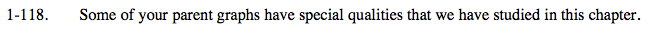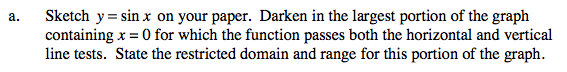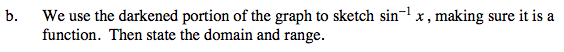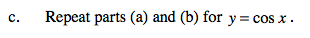### Home > CALC > Chapter 1 > Lesson 1.3.2 > Problem1-118

1-118.Graph the sin curve.

The domain is all of the x-values included in the graph and the range is all of the y-values that are included in the graph. Remember when looking at the graph that π equals about 3.1415.Graph the inverse of y = sin(x) by reflecting the graph of sin(x) across the line y = x.Repeat the steps in parts a and b using y = cos(x).

Use the eTool below to view the graphs.
Click on the link to the right to view the full version of the eTool. Calc 1-118 HW eTool